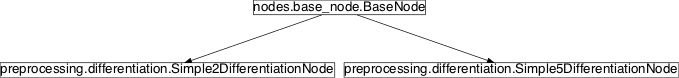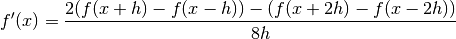# differentiation¶

## Module: missions.nodes.preprocessing.differentiation¶

Differentiate TimeSeries channel wise

Author: Marc Tabie (mtabie@informatik.uni-bremen.de) 2010/01/20

Inheritance diagram for pySPACE.missions.nodes.preprocessing.differentiation:## Class Summary¶

 Simple2DifferentiationNode([datapoints, ...]) Calculate difference to previous time point to generate new TimeSeries Simple5DifferentiationNode([datapoints, ...]) Calculate smoothed derivative using 5 time points

## Classes¶

### Simple2DifferentiationNode¶

class pySPACE.missions.nodes.preprocessing.differentiation.Simple2DifferentiationNode(datapoints=None, moving_window_length=1, keep_number_of_samples=False, **kwargs)[source]

Calculate difference to previous time point to generate new TimeSeries

Parameters
datapoints: The indices of the data points that are used as features, If None, all data points are used. (optional, default: None)

Exemplary Call

-
node : diff2

POSSIBLE NODE NAMES:

• diff2
• Simple2DifferentiationNode
• Simple2Differentiation
POSSIBLE INPUT TYPES:

• TimeSeries

Class Components Summary

 _execute(x) f’ = (f(x+h)-f(x)) input_types
__init__(datapoints=None, moving_window_length=1, keep_number_of_samples=False, **kwargs)[source]
_execute(x)[source]

f’ = (f(x+h)-f(x))

input_types = ['TimeSeries']

### Simple5DifferentiationNode¶

class pySPACE.missions.nodes.preprocessing.differentiation.Simple5DifferentiationNode(datapoints=None, moving_window_length=1, keep_number_of_samples=False, **kwargs)[source]

Calculate smoothed derivative using 5 time pointsFurther smoothing functions are available, but seemingly not necessary, because we have already a smoothing of the signal when doing the subsampling. Dividing by 8h is omitted, because it is just multiplication with the same scalar each time.

Parameters
datapoints: The indices of the data points that are used as features, If None, all data points are used. (optional, default: None)

Exemplary Call

-
node : diff5

POSSIBLE NODE NAMES:

• diff5
• Simple5Differentiation
• Simple5DifferentiationNode
POSSIBLE INPUT TYPES:

• TimeSeries

Class Components Summary

 _execute(x) Calculate derivative input_types
__init__(datapoints=None, moving_window_length=1, keep_number_of_samples=False, **kwargs)[source]
_execute(x)[source]

Calculate derivativeinput_types = ['TimeSeries']